# Flux Balance Analysis

## INTRODUCTION

In this tutorial, Flux Balance Analysis (FBA) is introduced using the E. coli core model, with functions in the COBRA Toolbox v3.0 .
Flux balance analysis is a solution to the optimisation problemwhere c is a vector of linear objective coefficients, S is an m times n matrix of stoichiometric coefficients for m molecular species involved in n reactions.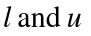are n times 1 vectors that are the lower and upper bounds on the n times 1 variable vector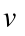of reaction rates (fluxes). The optimal objective value is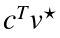is always unique, but the optimal vector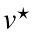is usually not unique.
In summary, the data is {c,S,l,u} and the variable being optimised is v.

< 1 hrs

## E. coli core model

A map of the E. coli core model is shown in Figure 1.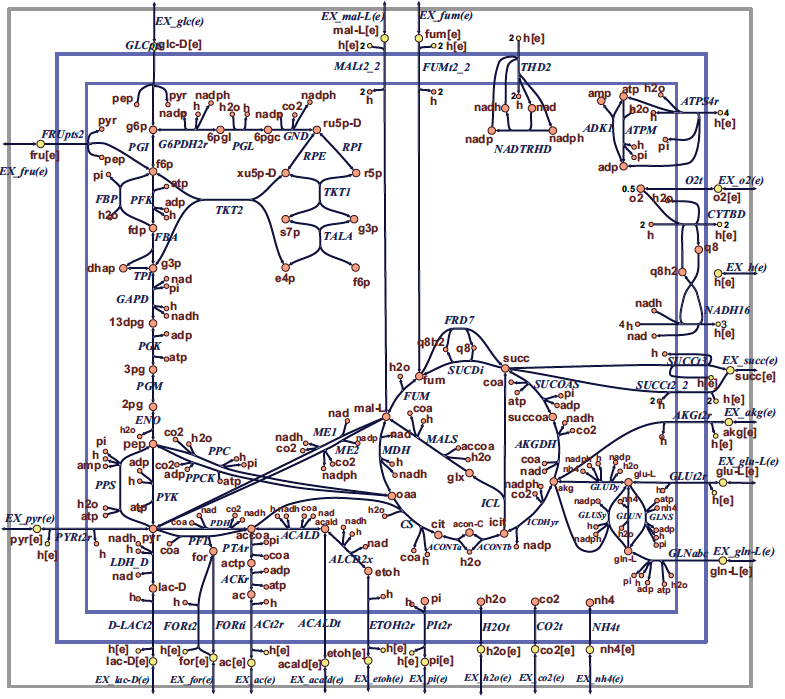Figure 1 Map of the core E. coli metabolic network. Orange circles represent cytosolic metabolites, yellow circles represent extracellular metabolites, and the blue arrows represent reactions. Reaction name abbreviations are uppercase (blue) and metabolite name abbreviations are lowercase (rust colour). This flux map was drawn using SimPheny and edited for clarity with Adobe Illustrator.

## MATERIALS - EQUIPMENT SETUP

Please ensure that all the required dependencies (e.g. , git and curl) of The COBRA Toolbox have been properly installed by following the installation guide here. Please ensure that the COBRA Toolbox has been initialised (tutorial_initialize.mlx) and verify that the pre-packaged LP and QP solvers are functional (tutorial_verify.mlx).

## Load E. coli core model

The most appropriate way to load a model into The COBRA Toolbox is to use the readCbModel function.
fileName = 'ecoli_core_model.mat';
if ~exist('modelOri','var')
end
%backward compatibility with primer requires relaxation of upper bound on
%ATPM
modelOri = changeRxnBounds(modelOri,'ATPM',1000,'u');
model = modelOri;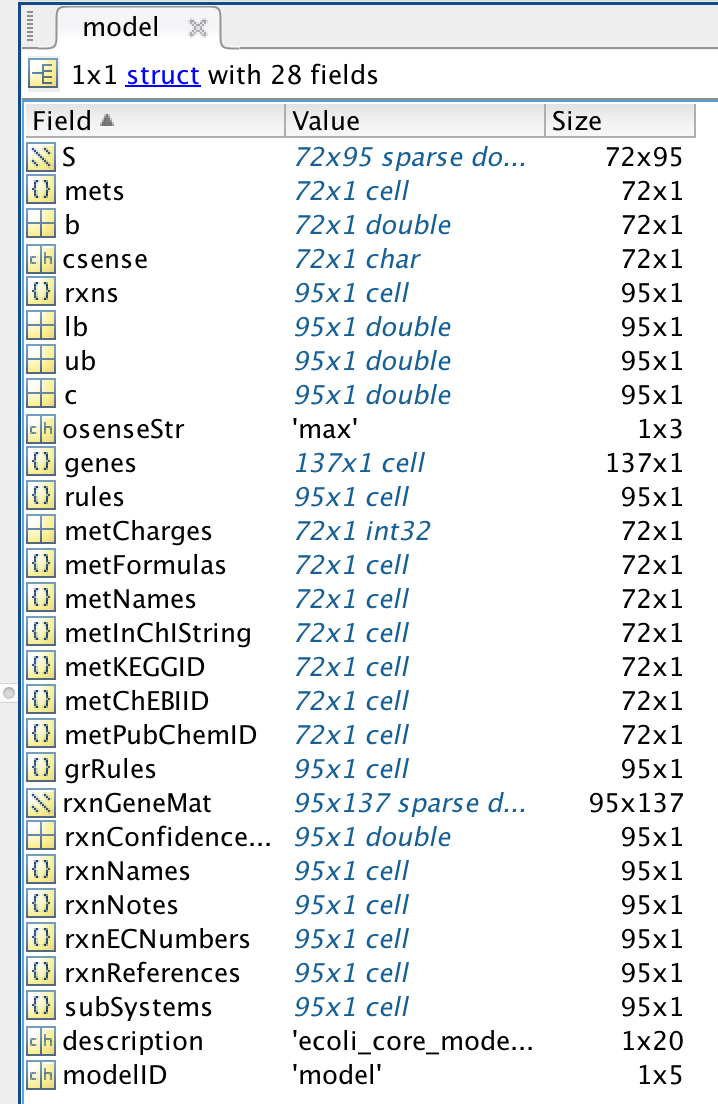The meaning of each field in a standard model is defined in the standard COBRA model field definition.
In general, the following fields should always be present:
• S, the stoichiometric matrix
• mets, the identifiers of the metabolites
• b, Accumulation (positive) or depletion (negative) of the corresponding metabolites. 0 Indicates no concentration change.
• csense, indicator whether the b vector is a lower bound ('G'), upper bound ('L'), or hard constraint 'E' for the metabolites.
• rxns, the identifiers of the reactions
• lb, the lower bounds of the reactions
• ub, the upper bounds of the reactions
• c, the linear objective
• genes, the list of genes in your model
• rules, the Gene-protein-reaction rules in a computer readable format present in your model.
• osenseStr, the objective sense either 'max' for maximisation or 'min' for minimisation

## Checking the non-trivial constraints on a model

#### Hint: printConstraints

printConstraints(model,-1000,1000)
MinConstraints: ATPM 8.39 EX_glc(e) -10 maxConstraints:

## Example 1: Calculating growth rates

Growth of E. coli on glucose can be simulated under aerobic conditions.

#### Hint: changeRxnBounds, changeObjective, optimizeCbModel, printFluxVector

To set the maximum glucose uptake rate to 18.5 mmol gDW-1 hr-1 (millimoles per gram dry cell weight per hour, the default flux units used in the COBRA Toolbox), enter:
model = changeRxnBounds(model,'EX_glc(e)',-18.5,'l');
This changes the lower bound ('l') of the glucose exchange reaction to -18.5, a biologically realistic uptake rate. By convention, exchange reactions are written as export reactions (e.g. ‘glc[e] <==>’), so import of a metabolite is a negative flux.
To allow unlimited oxygen uptake, enter:
model = changeRxnBounds(model,'EX_o2(e)',-1000,'l');
By setting the lower bound of the oxygen uptake reaction to such a large number, it is practically unbounded. Next, to ensure that the biomass reaction is set as the objective function, enter:
model = changeObjective(model,'Biomass_Ecoli_core_N(w/GAM)-Nmet2');
The default behaviour of the function optimizeCbModel is to conduct flux balance analysis on a model structure. This function is the most powerful function in the COBRA toolbox and has many optional inputs and outputs but for a straightforward flux balance analysis, the following fields of a model are the required input:
% model: (the following fields are required - others can be supplied)
%
% * S - m x 1 Stoichiometric matrix
% * c - n x 1 Linear objective coefficients
% * lb - n x 1 Lower bounds
% * ub - n x 1 Upper bounds
To perform FBA with maximization of the biomass reaction as the objective, enter:
FBAsolution = optimizeCbModel(model,'max')
FBAsolution = struct with fields:
full: [95×1 double] obj: 1.6531 rcost: [95×1 double] dual: [72×1 double] slack: [72×1 double] solver: 'glpk' algorithm: 'default' stat: 1 origStat: 5 time: 0.0280 basis: [] f: 1.6531 x: [95×1 double] v: [95×1 double] w: [95×1 double] y: [72×1 double] s: [72×1 double]

#### Hint: help optimizeCbModel

There are many outputs fields in the FBAsolution structure, but the key ones for any FBA solution are
% solution: solution object:
% * f - Objective value
% * v - Reaction rates (Optimal primal variable, legacy FBAsolution.x)
% * stat - Solver status in standardized form
FBAsolution.f gives the value of the objective function, which should be 1.6531 mmol gDW-1 hr-1
FBAsolution.f
ans = 1.6531
This is the same as
model.c'*FBAsolution.v
ans = 1.6531
This means that the model predicts a growth rate of 1.6531 hr-1. Inspection of the flux distribution vector FBAsolution.v shows that there is high flux in the glycolysis, pentose phosphate, TCA cycle, and oxidative phosphorylation pathways, and that no organic by-products are secreted (Figure 2a).
FBAsolution.v gives the optimal flux vector
FBAsolution.v
ans = 95×1
0 0 0.0000 10.3657 10.3657 0 0 8.5822 0 0
Inspection of the flux distribution is more convenient with the printFluxVector function
fluxData = FBAsolution.v;
nonZeroFlag = 1;
printFluxVector(model, fluxData, nonZeroFlag)
ACONTa 10.37 ACONTb 10.37 AKGDH 8.582 ATPM 8.39 ATPS4r 80.61 Biomass_Ecoli_core_N(w/GAM)-Nmet2 1.653 CO2t -40.65 CS 10.37 CYTBD 77.48 ENO 26.84 EX_co2(e) 40.65 EX_glc(e) -18.5 EX_h2o(e) 52.69 EX_h(e) 33.16 EX_nh4(e) -9.014 EX_o2(e) -38.74 EX_pi(e) -6.081 FBA 13.56 FUM 8.582 G6PDH2r 9.881 GAPD 29.31 GLCpts 18.5 GLNS 0.4227 GLUDy -8.591 GND 9.881 H2Ot -52.69 ICDHyr 10.37 MDH 8.582 NADH16 68.9 NH4t 9.014 O2t 38.74 PDH 16.56 PFK 13.56 PGI 8.28 PGK -29.31 PGL 9.881 PGM -26.84 PIt2r 6.081 PPC 4.737 PYK 2.744 RPE 5.399 RPI -4.482 SUCDi 8.582 SUCOAS -8.582 TALA 2.998 TKT1 2.998 TKT2 2.401 TPI 13.56

## Example 2: Display an optimal flux vector on a metabolic map

#### Hint: drawFlux

outputFormatOK = changeCbMapOutput('matlab');
options.zeroFluxWidth = 0.1;
options.rxnDirMultiplier = 10;
drawFlux(map, model, FBAsolution.v, options);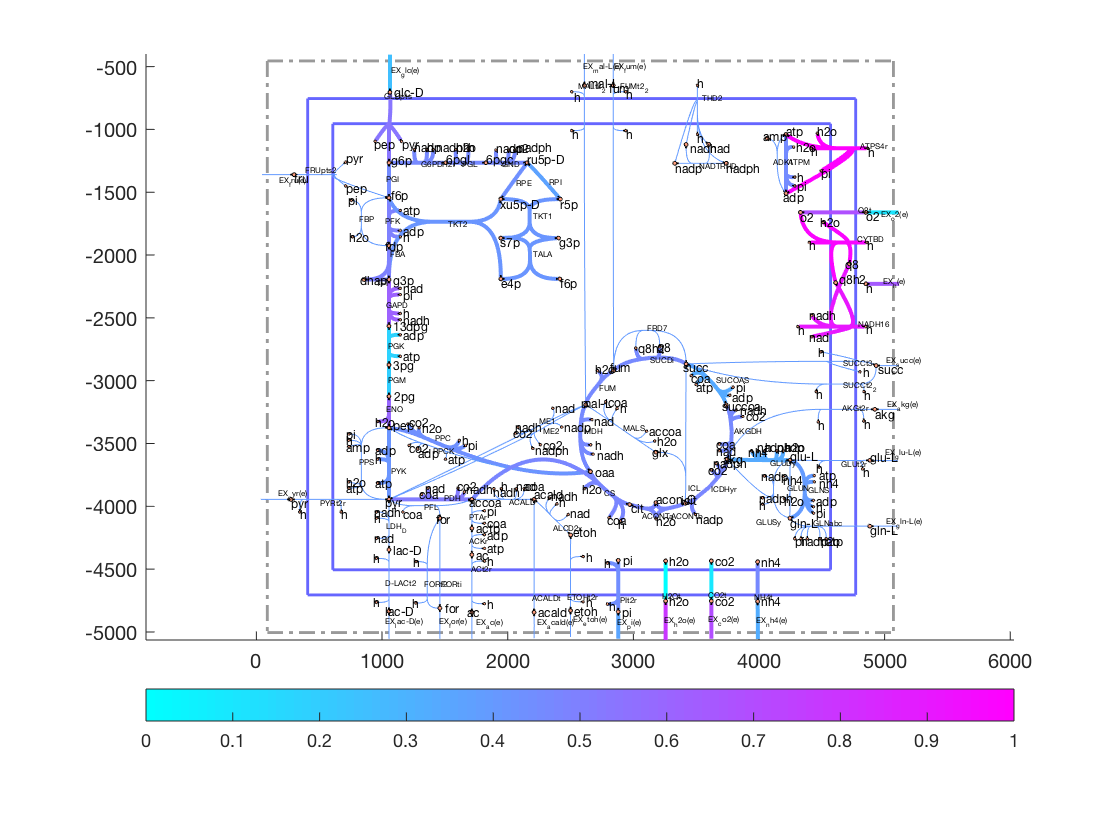## Example 3: Anerobic growth

Growth of E. coli on glucose can be simulated under anaerobic conditions.

#### Hint: changeRxnBounds

Next, the same simulation is performed under anaerobic conditions. With the same model:
model = changeRxnBounds(model,'EX_o2(e)',0,'l');
The lower bound of the oxygen exchange reaction is now 0, so oxygen may not enter the system. When optimizeCbModel is used as before, the resulting growth rate is now much lower, 0.4706 hr-1.
FBAsolution2 = optimizeCbModel(model,'max');
FBAsolution2.f
ans = 0.4706

#### Hint: printFluxVectordrawFlux

The flux distribution shows that oxidative phosphorylation is not used in these conditions, and that acetate, formate, and ethanol are produced by fermentation pathways (Figure 2b). Inspection of both flux vectors for comparison:
fluxData = [FBAsolution.v,FBAsolution2.v];
nonZeroFlag = 1;
excFlag = 1;
printFluxVector(model, fluxData, nonZeroFlag, excFlag)
Biomass_Ecoli_core_N(w/GAM)-Nmet2 1.653 0.4706 EX_ac(e) 0 15.17 EX_co2(e) 40.65 -0.8408 EX_etoh(e) 0 14.67 EX_for(e) 0 32.12 EX_glc(e) -18.5 -18.5 EX_h2o(e) 52.69 -12.09 EX_h(e) 33.16 56.73 EX_nh4(e) -9.014 -2.566 EX_o2(e) -38.74 0 EX_pi(e) -6.081 -1.731
drawFlux(map, model, FBAsolution2.v, options);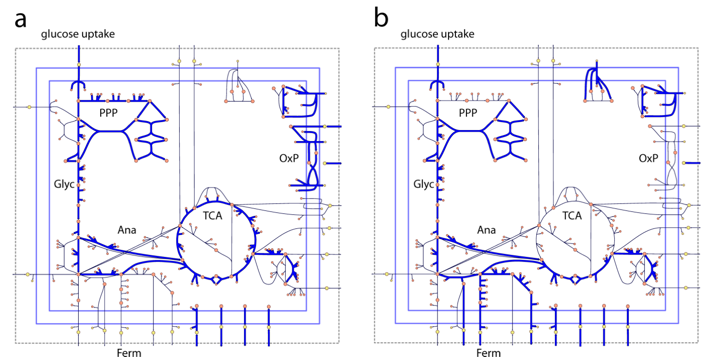Figure 2 Flux vectors computed by FBA can be visualized on network maps. In these two examples, the thick blue arrows represent reactions carrying flux, and the thin black arrows represent unused reactions. These maps show the state of the E. coli core model with maximum growth rate as the objective (Z) under aerobic (a) and anaerobic (b) conditions. Reactions that are in use have thick blue arrows, while reactions that carry 0 flux have thin black arrows. The metabolic pathways shown in these maps are glycolysis (Glyc), pentose phosphate pathway (PPP), TCA cycle (TCA), oxidative phosphorylation (OxP), anaplerotic reactions (Ana), and fermentation pathways (Ferm).

## Example 4: Growth on alternate substrates

Just as FBA was used to calculate growth rates of E. coli on glucose, it can also be used to simulate growth on other substrates. The core E. coli model contains exchange reactions for 13 different organic compounds, each of which can be used as the sole carbon source under aerobic conditions.

#### Hint: changeRxnBounds

Before trying out new boundary conditions on a model, make sure one's starting point is appropriate.
model = modelOri;
For example, to simulate growth on succinate instead of glucose, first use the changeRxnBounds function to set the lower bound of the glucose exchange reaction (EX_glc(e)) to 0.
model = changeRxnBounds(model,'EX_glc(e)',0,'l');
Then use changeRxnBounds to set the lower bound of the succinate exchange reaction (EX_succ(e)) to -20 mmol gDW-1 hr-1 (an arbitrary uptake rate).
model = changeRxnBounds(model,'EX_succ(e)',-20,'l');
As in the glucose examples, make sure that the biomass reaction is set as the objective (the function checkObjective can be used to identify the objective reaction(s)),
checkObjective(model);
summaryT = 23×5 table
CoefficientMetabolitemetIDReactionRxnID
1-1.49603pg[c]3Biomass_Ecoli_core_N(w/GAM)-Nmet213
2-3.7478accoa[c]10Biomass_Ecoli_core_N(w/GAM)-Nmet213